Next: VoronoiRatio Up: Physical/Computational parameters Previous: rstretch: or   Contents

### CorrectVoronoi: Boolean

Typical triangulations will always contain degenerate triangles in which one of the angles are close to or greater than 90 degrees. For near-right neighboring triangles, the distance between their Voronoi points can be quite small (zero when two right triangles abut one another), while for obtuse triangles, the Voronoi point lies outside the triangle. This can be corrected in one of two ways.

In the first method, CorrectVoronoi is 1, and the Voronoi points are corrected if they are too close to each other relative to the distance between the centroids of the neighboring triangles. If triangle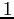and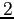have centroids defined by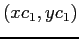and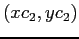and Voronoi points defined by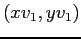and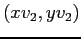, then the distance between the centroids is given by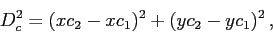and the distance between the Voronoi points is given by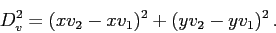The Voronoi points are corrected if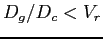, where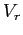is the VoronoiRatio parameter, and if this is the case, they are updated with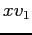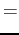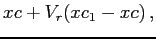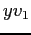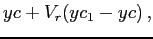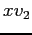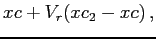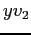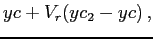where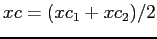and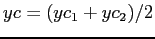. Using this correction methodology, setting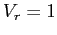moves the Voronoi points to the centroids of the cells.

In the second method, CorrectVoronoi is set to -1 and triangles with angles greater than or equal to the angle defined by VoronoiRatio are corrected by moving the Voronoi point to the triangle centroid. While the centroid is guaranteed to be inside the triangle and the distance between centroids is on the same order as the triangle edge lengths, the disadvantage is that the Voronoi edge connecting the Voronoi points is no longer perpendicular to the Delaunay edges. This reduces the accuracy of the gradients defined by differences between values at Voronoi points because it is assumed that the Voronoi-Delaunay intersection is orthogonal.Next: VoronoiRatio Up: Physical/Computational parameters Previous: rstretch: or   Contents
2014-08-06